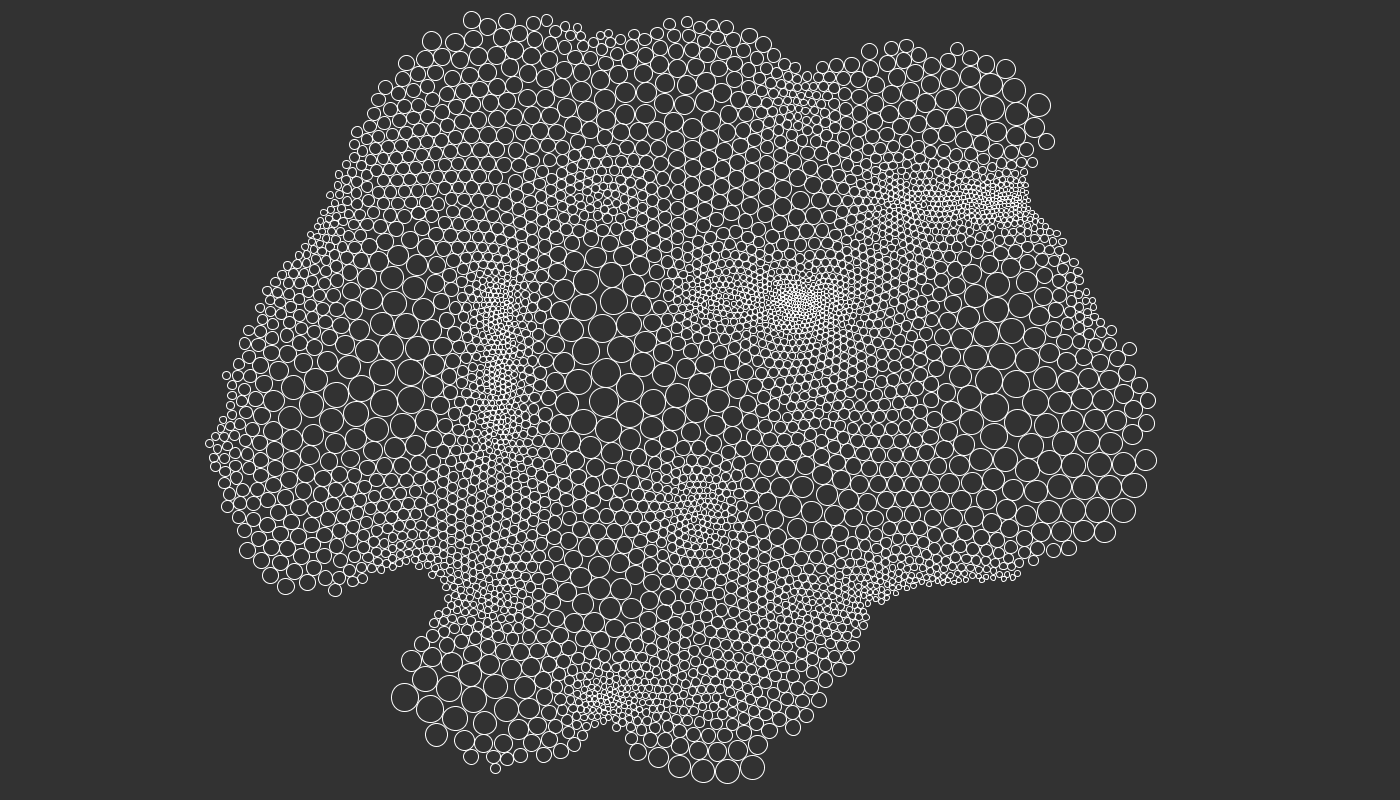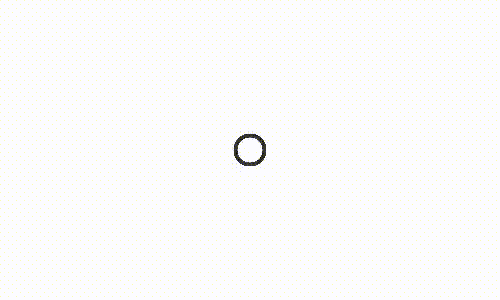Controlled Circle Packing with Processing

How to implement a controlled circle packing algorithm with ProcessingToday we will implement a circle packing algorithm using Processing. The inspiration for this tutorial came from this YouTube video, where Grasshopper was used. Also this time I have to thank Entagma for giving useful hints on how to achieve the result.

We are calling this controlled circle packing as opposed to random circle packing (here an example of the latter), where we randomly try to fit new circles inside the space left. In our case the circles will try to find by themselves their optimal/sub-optimal position by moving inside the given space.

The algorithm goes like this:

• If two circles are overlapping, move them apart;
• If a circle is not overlapping with any other circle, zero its velocity, hence stop its motion.

Also in this case, my starting point was the flocking algorithm written by Daniel Shiffman in the way they we revisited it on our Differential line growth with Processing tutorial.

We will then have a `Circle` class storing the information about position, velocity, acceleration and radius of each circle:

```class Circle {

PVector position;
PVector velocity;
PVector acceleration;

Circle(float x, float y) {
acceleration = new PVector(0, 0);
velocity = PVector.random2D();
position = new PVector(x, y);
}

void applyForce(PVector force) {
}

void update() {
acceleration.mult(0);
}

void display() {
}
}```

We will then create a `Pack` class which will perform the algorithm. Inside the `Pack` class, first of all we populate the array of circles with the` initiate()` method. The array can eventually be expanded while running the program with the `addCircle()` method. The main method of the class if `run()`, where the following operations are conducted for each circle:

• With `checkBorders()` we make sure that the circles don’t go out of the canvas’ borders by bouncing them back when this happens;
• With `checkCirclePosition()` we zero the velocity of the circle once we have verified that it is not overlapping with any other circle;
• With `applySeparationForcesToCircle()` we check the position of the circle against the position of all the other circles and if there is an overlapping we apply a repulsing force to both;
• Finally, we render the circle with `displayCircle()`;
```class Pack {
ArrayList<Circle> circles;

float max_speed = 1;
float max_force = 1;

Pack() {
initiate();
}

void initiate() {
circles = new ArrayList<Circle>();
for (int i = 0; i < 750; i++) {
}
}

}

void run() {

PVector[] separate_forces = new PVector[circles.size()];
int[] near_circles = new int[circles.size()];

for (int i=0; i<circles.size(); i++) {
checkBorders(i);
checkCirclePosition(i);
applySeparationForcesToCircle(i, separate_forces, near_circles);
displayCircle(i);
}
}

void checkBorders(int i) {
Circle circle_i=circles.get(i);
{
circle_i.velocity.x*=-1;
circle_i.update();
}
{
circle_i.velocity.y*=-1;
circle_i.update();
}
}

void checkCirclePosition(int i) {

Circle circle_i=circles.get(i);

for (int j=i+1; j<=circles.size(); j++) {

Circle circle_j = circles.get(j == circles.size() ? 0 : j);

int count = 0;

float d = PVector.dist(circle_i.position, circle_j.position);

count++;
}

// Zero velocity if no neighbours
if (count == 0) {
circle_i.velocity.x = 0.0;
circle_i.velocity.y = 0.0;
}
}
}

void applySeparationForcesToCircle(int i, PVector[] separate_forces, int[] near_circles) {

if (separate_forces[i]==null)
separate_forces[i]=new PVector();

Circle circle_i=circles.get(i);

for (int j=i+1; j<circles.size(); j++) {

if (separate_forces[j] == null)
separate_forces[j]=new PVector();

Circle circle_j=circles.get(j);

PVector forceij = getSeparationForce(circle_i, circle_j);

if (forceij.mag()>0) {
separate_forces[j].sub(forceij);
near_circles[i]++;
near_circles[j]++;
}
}

if (near_circles[i]>0) {
separate_forces[i].div((float)near_circles[i]);
}

if (separate_forces[i].mag() >0) {
separate_forces[i].setMag(max_speed);
separate_forces[i].sub(circles.get(i).velocity);
separate_forces[i].limit(max_force);
}

PVector separation = separate_forces[i];

circles.get(i).applyForce(separation);
circles.get(i).update();
}

PVector getSeparationForce(Circle n1, Circle n2) {
PVector steer = new PVector(0, 0, 0);
float d = PVector.dist(n1.position, n2.position);
PVector diff = PVector.sub(n1.position, n2.position);
diff.normalize();
diff.div(d);
}
return steer;
}

void displayCircle(int i) {
circles.get(i).display();
}
}```

Here we have a fixed circles’ radius:Finally, like in the image at the top of the article, we can make the radius adapt to some value, for example a Perlin noise field. For this purpose I’ve created a new method inside the `Circle` class and one inside the `Pack` class:

```class Pack {

[...]

void run() {

PVector[] separate_forces = new PVector[circles.size()];
int[] near_circles = new int[circles.size()];

for (int i=0; i<circles.size(); i++) {
checkBorders(i);
checkCirclePosition(i);
applySeparationForcesToCircle(i, separate_forces, near_circles);
displayCircle(i);
}
}

}

[...]
}

class Circle {

[...]

radius = 2 + noise(position.x*0.01, position.y*0.01) * 50;
}

}```Now I am wondering how this could be use to make something out of CNC or 3D printing… material for the next tutorial 😉

Code!1.JPFrancoia says:

Hi, very nice tutorial. I’m going to try to port your code to python. I have a question though: my need is not to do circle packing, but rather circle fitting: I have N circles (they can have different radius), and my goal is to fit them in a big circle, so that the distance between them AND the big circle’s edge is maximised. Would your code be able to do that ? Because from what I understand, the force pushing the circles away from each other is set to zero as soon as the circles don’t overlap. Cheers

1.Alberto Giachino says:

Hi, thank you! I think that this code could be a good starting point but you’ll have to do some tweaking. An idea is to add the separation force for every particle proportionally to their distance and sizes, plus the separation force from the big circle. At a certain point the system should reach some sort of equilibrium. Let me know how it goes.

1.Alberto Giachino says:

My Python level is something like 30 minutes of tutorial 🙂 I had a look at your question, for now I can tell you that definitely run() runs multiple times: the draw() function is a drawing loop that runs 30-60 times per seconds. If you don’t have something like this in your code you will have to use some while loop and then implement a function that detects when the system has reached the equilibrium (e.g. all circles are still) to stop the loop. By the way have you heard about Python Processing? http://py.processing.org/

1.JPFrancoia says:

The porting/modification of your code is done. Now I can pack circles in a big circle 🙂 (check the question on SO).
Now I’ll start doing what I initially wanted to do.

2.Alberto Giachino says:

Nice, happy to hear that:)